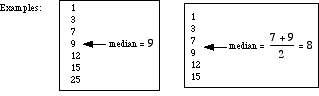index: click on a letter A B C D E F G H I J K L M N O P Q R S T U V W X Y Z A to Z index index: subject areas numbers & symbols sets, logic, proofs geometry algebra trigonometry advanced algebra & pre-calculus calculus advanced topics probability & statistics real world applications multimedia entrieswww.mathwords.com about mathwords website feedback

 Median of a Set of Numbers The median of a set of numbers is the value for which half the numbers are larger and half are smaller. If there are two middle numbers, the median is the arithmetic mean of the two middle numbers. Note: The median is a good choice to represent the center of a distribution when the distribution is skewed or has outliers.See also